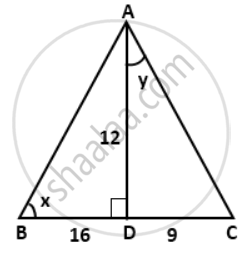# In the given figure, AD is perpendicular to BC. Find: 15 tan y - Mathematics

Sum

In the given figure, AD is perpendicular to BC. Find: 15 tan y#### Solution∴ AB2
= AB2 + BD2
= 122 + 162
= 144 + 256
= 400
⇒ AB = 20cm
∴ AC2
= 122 + 92
= 144 + 81
= 225
⇒ AC = 15cm
15 tan y

= 15 xx "CD"/"AD"

= 15 xx (9)/(12)

= (45)/(4).

Concept: Reciprocal Relations
Is there an error in this question or solution?

#### APPEARS IN

Frank Class 9 Maths ICSE
Chapter 26 Trigonometrical Ratios
Exercise 26.1 | Q 20.2